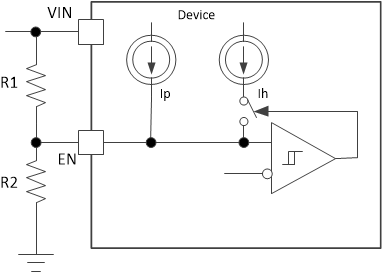SLUSDX1A September   2020  – August 2021

PRODUCTION DATA

1. Features
2. Applications
3. Description
4. Revision History
5. Pin Configuration and Functions
6. Specifications
7. Detailed Description
1. 7.1 Overview
2. 7.2 Functional Block Diagram
3. 7.3 Feature Description
4. 7.4 Device Functional Modes
8. Application and Implementation
1. 8.1 Application Information
2. 8.2 Typical Application
9. Power Supply Recommendations
10. 10Layout
11. 11Device and Documentation Support
12. 12Mechanical, Packaging, and Orderable Information

• DRL|8

### 7.3.6 Precise Enable and Adjusting Undervoltage Lockout

The EN pin provides electrical on and off control for the device. When the EN pin voltage exceeds the threshold voltage, the device begins operation. If the EN pin voltage is pulled below the threshold voltage, the regulator stops switching and enters the shutdown mode.

The EN pin has an internal pullup source current, which allows the user to float the EN pin to enable the device. If an application requires control of the EN pin, use external control logic interface to the EN the pin like the open-drain or open-collector output logic.

The device implements internal undervoltage-lockout (UVLO) circuitry on the VIN pin. The device is disabled when the VIN pin voltage falls below the internal VIN UVLO threshold. The internal VIN UVLO threshold has a hysteresis of 400 mV.

If an application requires a higher UVLO threshold on the VIN pin, the EN pin can be configured as shown in Figure 7-3. When using the external UVLO function, setting the hysteresis at a value greater than 400 mV is recommended.

The EN pin has a small pullup current, Ip, which sets the default state of the EN pin to enable when no external components are connected. The pullup current is also used to control the voltage hysteresis for the external UVLO function because it increases by Ih when the EN pin crosses the enable threshold. Use Equation 3 and Equation 4 to calculate the values of R1 and R2 for a specified UVLO threshold. Once R1 and R2 have settled down, the EN voltage can be calculated by Equation 5, which must be lower than 5.5 V with the maximum VIN.

Equation 3. ${R}_{1}=\frac{{V}_{START}\bullet \frac{{V}_{EN_FALL}}{{V}_{EN_RISE}}-{V}_{STOP}}{{I}_{p}\left(1-\frac{{V}_{EN_FALL}}{{V}_{EN_RISE}}\right)+{I}_{h}}$
Equation 4. ${R}_{2}=\frac{{R}_{1}\bullet {V}_{EN_FALL}}{{V}_{STOP}-{V}_{EN_FALL}+{R}_{1}\bullet \left({I}_{p}+{I}_{h}\right)}$
Equation 5. ${V}_{EN}=\frac{{R}_{2}\bullet {V}_{IN}+{R}_{1}{R}_{2}\left({I}_{p}+{I}_{h}\right)}{{R}_{1}+{R}_{2}}$

where

• Ip = 1.2 µA
• Ih = 3.1 µA
• VEN_FALL = 1.15 V
• VEN_RISE = 1.01 V
• VSTART, the expected input voltage enabling the device
• VSTOP, the expected input voltage disabling the deviceFigure 7-3 Adjustable VIN Undervoltage Lockout